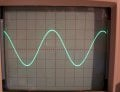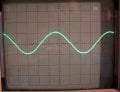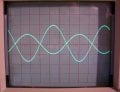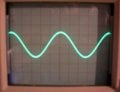# Choosing the values of the resistors

Status
Not open for further replies.

#### hazim

Joined Jan 3, 2008
434
I have just finished the BS degree in Electronics Engineering. I can understand the attached circuit of course.. I learned to analyze all the classes of the power amplifiers...etc. We didn't learned to design practical circuits including power amplifiers, I don't know if this is normal or what.
Anyway, I attached this circuit now to ask you about the values chose for the resistors mainly, and the capacitors.

Why to choose 27kΩ and not 22k? or even 1k or 100k?? the same for the other resistors but mainly the 27k and the 390k.Hazim

#### Audioguru

Joined Dec 20, 2007
11,248
The voltage gain of the amplifier is determined by the ratio of the 2.2k resistor and the 1k resistor. This calculated gain is reduced by negative feedback through the 390k resistor cancelling some of the input signal since they have the opposite phase.
The 27k resistor reduces the gain some more but increases the input impedance.

You should learn a simulation program.
I simulated the circuit and changed resistor values and reduced the input level so that the output level stays nearly the same.

The capacitor values are calculated with the resistors they feed for a certain low frequency cutoff. 1 divided by 2 pi R x C.

#### Attachments

•hazim

#### hazim

Joined Jan 3, 2008
434
I downloaded LTSpice and used it. It seems better than Multisim in many things.. I used to use Multisim.
Ok, I changed the values of the resistors and capacitors and saw what happens with the signal... I can understand it better now. Tomorrow I'll post a new thread with another circuit, a more complicated one, and I will try to understand it entirely with the help of this forum's capable members.

Thanks
Hazim

#### Jony130

Joined Feb 17, 2009
5,316
In general the Q3 Ic current must be 5..20 times larger then I_load/ hfe_min_Q4
And 390K we choose that voltage on emitter of a Q4, Q5 equals 0.5*Vcc

•hazim

#### zhgart

Joined Dec 28, 2009
19
why not 22k, but choose 27k and 390k instead of 360k?

#### zhgart

Joined Dec 28, 2009
19
first, you have to be aware of the pre amplifier is a current feedback amplifier, the amplifier has an advantage of less currrent distortion and easy match with front circuit.

#### zhgart

Joined Dec 28, 2009
19
first, you have to be aware of the pre amplifier is a current feedback amplifier, the amplifier has an advantage of less currrent distortion and easy match with front circuit.
the max output power is 4.5^2/8=20.25/8=1.5w and I=0.3A, if Q4,5 beta is about 100 you will get Icq3=3ma, if its beta=100, Ib=30uA. wherea 390k/100=4k, 1kx100=100k, so you can choose either 27k or 22k or other values, like 30k,10k..
but 390k will change with its value depend on magnitude.

#### zhgart

Joined Dec 28, 2009
19
if your front amplifier is more output, you can choose more than , say 100k in place of 27k.
how much is that output? 4.5/15= 0.3v, and inner resistance will be U`-0.3/30uA, suppose U=1v, r<=700/30=23k, so you can also choos 22k. no problem.

•hazim

#### hobbyist

Joined Aug 10, 2008
890
Hi
(DISCLAIMER):

My main desire in contributing to this forum, is to help those who are just starting out in this field, with the very basics in transistor circuit design. I myself am NOT a professional, NOR do I profess to be, nor do I work in this field, I am ONLY a Hobbyist, with the enjoyment of learning in this field, and applying it to circuit design and prototyping circuits for my own personal projects.
So my aim in this forum is to breakdown the barrier mindset, That says " designing a circuit from scratch is so formidable".
By showing how to design a working circuit, from a beginners basic point of view.
I consider myself a basic beginner in this field, so all I share is from a beginners perspective only.
If I can show you how to design a working circuit if ONLY to give you confidence when you design and build it yourself, to break the formidable barrier,
I will be glad to have helped, from there it is up to you to take that confidence to the next level, into practical professional design techniques.
I am only trying to build beginners confidence in giving hands on experiance in designing a workable circuit.
----------------------------------------------------------------------------------------------------------------------------------------------------------

I built the original circuit and here are the results. I used a 1Khz signal freq. and Load is a 8.2 ohm resistor.

VEQ4= 5.11v

VBQ4= 5.73v

Resulting waveform:

INPUT WAVEFORM @ 1v. pk.OUTPUT WAVEFORM @ 0.5v. pk. (Notice the very small crossover distortion at the zero volt line.)Both waveforms, (INPUT is larger due to the 27K series dropping resistor.)From the data above it can be determined that this was NOT designed to be a voltage amplifier, BUT a POWER amp, to deliver maximaum volt. and current to a
very LOW load impedance, of 8ohms.
------------------------------------------------------------------------------------------------------------------------------------------------------
My (basic beginners) way of designing the same circuit:

Design procedures:

VCC= 9v.

VEQ4 = (VCC / 2) = 4.5v

VBQ4 = (VEQ4 + Vbe)= 5.2v

The VBQ5 needs to be lower by (VbeQ4 + VbeQ5) = 1.4v

So VBQ5 will need 2 diode voltage drops to attain this, hence the reason for using 2 diodes in this circuit.

VBQ5= (VEQ5 - Vbe)= 3.8v ......... which = (VBQ4 - 0.7v - 0.7v)

For starters I'll choose 10mA, for IEQ4
IEQ4= 10mA.

Iload pk-pk= (IEQ4 / 1.2) what that shows is load current pk-pk is 20% lower than emitter current of Q4,Q5.

IbQ4= (IEQ4 / Beta min.) Data sheet shows Beta min. 100 @ 10mA.
IbQ4= 100uA.

Now the collector current ICQ3, will bias the Q4,Q5 network, this current needs to be at least 10 times greater than IBQ4.
ICQ3= (10 x IBQ4)= 1mA.

Top base resistor of Q4, call it RCQ3, = {(Vcc - VBQ4) / ICQ3}
RCQ3= 3.8K ohms. make it standard 3.9K ohms.

Now I will try to get some gain with this amp.... Av= (RC / RE) and REQ3= (RCQ3 / AvQ3)
So I'll choose AvQ3 to be 10, and see if it can be realized when I prototype it.
REQ3= 390 ohms.

VEQ3= (ICQ3 x REQ3)= 390mV.

VBQ3= (VEQ3 +Vbe)= 1.09v.

Now IBQ3 = (ICQ3 / Beta min)= 10uA.

The base resistor connected to Q3, call it RBQ3, is calculated as follows.
The top side connects to the output voltage which is 4.5v.
The bottom side connects to the base voltage of 1.09v.
So the volt drop across this resistor ,(VRBQ3),= (VEQ4 - VBQ3)= 3.41v.

Now RBQ3 can be calculated as (VRBQ3 / IBQ3)= 341K ohms, make it standard 330K ohms.
------------------------------------------------------------------------------------
Now values are follows: (KEEPING THE SAME 27K SERIES DROPPING RESISTOR IN PLACE)

IEQ4,Q5= 10mA.

RCQ3= 3.9K ohms

REQ3= 390 ohms

RBQ3= 330K ohms
-------------------------------------
Now prototype and test measurements:

VEQ4= 3.35v. (A little low from calculated value but may still work.) <<<WHY>>>(The calculated vbalue was 341K so a higher resistance here would raise the output voltage, I'm using 330K ohms, which means less VBQ4)

Input waveform:Output waveform:Now dropping the input until output is at 500mV pk like the first circuit:From the waveforms I have some amplification.
The input signal had to be dropped from the original circuit of 1v.
down to 400mV. To get the same output voltage of 500mV.

This is more of a voltage amplifier than a power amp, compared to the first circuit.
Mainly because I chose a small value of IEQ4 10mA, which I used to determine the amount of available output current.

But this shows, that a design can be implemented from various parameters.
The parameter I chose was choosing the emitter current, to dictate this design.

Other ways could be to choose a output voltage, or power output, or any number of ways.

This was to help people get a beginners basic concept of how to get a very small grasp at the what seems to be formidable resistor values in a circuit like this.

When you study this and then design and build your own working circuit, your confidence will sore, which may give you the desire to increase your knowledge to get you to the next level to design circuits in a professional way.

Have fun and gain the confidence:

Last edited:
•Sara Nicole, phill.oye and hazim

#### beenthere

Joined Apr 20, 2004
15,819

#### retched

Joined Dec 5, 2009
5,208

#### beenthere

Joined Apr 20, 2004
15,819
Good idea. If this seems to be a good place, we will leave this here as an example.

#### hazim

Joined Jan 3, 2008
434
Thank you hobbyist.. thank you all. In the university we learned to calculate the currents in circuit diagrams, we learned to make dc and ac analysis in the different power amplifier classes, find the power and efficiency... gain and frequency response..etc. From this thread I have learned that in order to design such a circuit (signal/power amp.) with transistors, I should follow the procedure of analyzing the circuit reversely, i.e. to start by determining the current or voltage needed at the output and go reversely in calculating the currents and voltages to finally find the resistor values#### shortbus

Joined Sep 30, 2009
9,328
hobbyist, Thank You for a great post! This is the best 'lesson' I've ever seen. Do you have more of this type of wisdom on other components that you would share?

Thanks again.

#### hobbyist

Joined Aug 10, 2008
890
hobbyist, Thank You for a great post! This is the best 'lesson' I've ever seen. Do you have more of this type of wisdom on other components that you would share?

Thanks again.

Thankyou,

This is knowledge that is CONTINUALLY shared by OTHERS on this forum,

I just took the time to break it down into more smaller bite size pieces, for our level of understanding, in the basic level,
that's why I can relate to novices in this field, because that's the level I choose to stay at in my working with transistor circuits, and sharing that through hands on experiance.

Thankyou...

#### R!f@@

Joined Apr 2, 2009
9,784
Hi
(DISCLAIMER):

My main desire in contributing to this forum, is to help those who are just starting out in this field, with the very basics in transistor circuit design. I myself am NOT a professional, NOR do I profess to be, nor do I work in this field, I am ONLY a Hobbyist, with the enjoyment of learning in this field, and applying it to circuit design and prototyping circuits for my own personal projects.
So my aim in this forum is to breakdown the barrier mindset, That says " designing a circuit from scratch is so formidable".
By showing how to design a working circuit, from a beginners basic point of view.
I consider myself a basic beginner in this field, so all I share is from a beginners perspective only.
If I can show you how to design a working circuit if ONLY to give you confidence when you design and build it yourself, to break the formidable barrier,
I will be glad to have helped, from there it is up to you to take that confidence to the next level, into practical professional design techniques.
I am only trying to build beginners confidence in giving hands on experiance in designing a workable circuit.
----------------------------------------------------------------------------------------------------------------------------------------------------------

I built the original circuit and here are the results. I used a 1Khz signal freq. and Load is a 8.2 ohm resistor.

VEQ4= 5.11v

VBQ4= 5.73v

Resulting waveform:

INPUT WAVEFORM @ 1v. pk.
View attachment 16902

OUTPUT WAVEFORM @ 0.5v. pk. (Notice the very small crossover distortion at the zero volt line.)

View attachment 16903
Both waveforms, (INPUT is larger due to the 27K series dropping resistor.)

View attachment 16904

From the data above it can be determined that this was NOT designed to be a voltage amplifier, BUT a POWER amp, to deliver maximaum volt. and current to a
very LOW load impedance, of 8ohms.
------------------------------------------------------------------------------------------------------------------------------------------------------
My (basic beginners) way of designing the same circuit:

Design procedures:

VCC= 9v.

VEQ4 = (VCC / 2) = 4.5v

VBQ4 = (VEQ4 + Vbe)= 5.2v

The VBQ5 needs to be lower by (VbeQ4 + VbeQ5) = 1.4v

So VBQ5 will need 2 diode voltage drops to attain this, hence the reason for using 2 diodes in this circuit.

VBQ5= (VEQ5 - Vbe)= 3.8v ......... which = (VBQ4 - 0.7v - 0.7v)

For starters I'll choose 10mA, for IEQ4
IEQ4= 10mA.

Iload pk-pk= (IEQ4 / 1.2) what that shows is load current pk-pk is 20% lower than emitter current of Q4,Q5.

IbQ4= (IEQ4 / Beta min.) Data sheet shows Beta min. 100 @ 10mA.
IbQ4= 100uA.

Now the collector current ICQ3, will bias the Q4,Q5 network, this current needs to be at least 10 times greater than IBQ4.
ICQ3= (10 x IBQ4)= 1mA.

Top base resistor of Q4, call it RCQ3, = {(Vcc - VBQ4) / ICQ3}
RCQ3= 3.8K ohms. make it standard 3.9K ohms.

Now I will try to get some gain with this amp.... Av= (RC / RE) and REQ3= (RCQ3 / AvQ3)
So I'll choose AvQ3 to be 10, and see if it can be realized when I prototype it.
REQ3= 390 ohms.

VEQ3= (ICQ3 x REQ3)= 390mV.

VBQ3= (VEQ3 +Vbe)= 1.09v.

Now IBQ3 = (ICQ3 / Beta min)= 10uA.

The base resistor connected to Q3, call it RBQ3, is calculated as follows.
The top side connects to the output voltage which is 4.5v.
The bottom side connects to the base voltage of 1.09v.
So the volt drop across this resistor ,(VRBQ3),= (VEQ4 - VBQ3)= 3.41v.

Now RBQ3 can be calculated as (VRBQ3 / IBQ3)= 341K ohms, make it standard 330K ohms.
------------------------------------------------------------------------------------
Now values are follows: (KEEPING THE SAME 27K SERIES DROPPING RESISTOR IN PLACE)

IEQ4,Q5= 10mA.

RCQ3= 3.9K ohms

REQ3= 390 ohms

RBQ3= 330K ohms
-------------------------------------
Now prototype and test measurements:

VEQ4= 3.35v. (A little low from calculated value but may still work.) <<<WHY>>>(The calculated vbalue was 341K so a higher resistance here would raise the output voltage, I'm using 330K ohms, which means less VBQ4)

Input waveform:

View attachment 16905

Output waveform:

View attachment 16906

Now dropping the input until output is at 500mV pk like the first circuit:
View attachment 16908

From the waveforms I have some amplification.
The input signal had to be dropped from the original circuit of 1v.
down to 400mV. To get the same output voltage of 500mV.

This is more of a voltage amplifier than a power amp, compared to the first circuit.
Mainly because I chose a small value of IEQ4 10mA, which I used to determine the amount of available output current.

But this shows, that a design can be implemented from various parameters.
The parameter I chose was choosing the emitter current, to dictate this design.

Other ways could be to choose a output voltage, or power output, or any number of ways.

This was to help people get a beginners basic concept of how to get a very small grasp at the what seems to be formidable resistor values in a circuit like this.

When you study this and then design and build your own working circuit, your confidence will sore, which may give you the desire to increase your knowledge to get you to the next level to design circuits in a professional way.

Have fun and gain the confidence:
Very good indeed

#### shortbus

Joined Sep 30, 2009
9,328
Thankyou,

This is knowledge that is CONTINUALLY shared by OTHERS on this forum,

I just took the time to break it down into more smaller bite size pieces, for our level of understanding, in the basic level,
that's why I can relate to novices in this field, because that's the level I choose to stay at in my working with transistor circuits, and sharing that through hands on experiance.

Thankyou...
Well I think it would be great if there was a place/forum here for just this kind of information. While the E-books here are good, the way you presented it makes more sense to a dummie like me. Thats why the screen name shortbus, I ride the that while learning electronics.#### retched

Joined Dec 5, 2009
5,208
Good teacher. You are a good writer. You may consider writing a book or interactive CD-rom for beginners.

#### hobbyist

Joined Aug 10, 2008
890
Good teacher. You are a good writer. You may consider writing a book or interactive CD-rom for beginners.

Thankyou for the nice complement.

Now and then I come up with a basic tutorial, for beginners, and I post them in the "General electronics chat section".

Status
Not open for further replies.